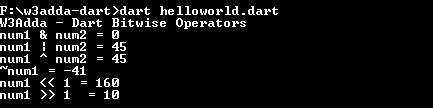# Dart Bitwise operators

In this tutorial you will learn about the Dart Bitwise operators and its application with practical example.

## Dart Bitwise Operators

Bitwise operator are used to perform bit level operation over its operand. Let A = 60; and B = 13;

Binary Equivalent:-

Operator Meaning Example Description
& Binary AND (A & B) It returns 12 which is 0000 1100
| Binary OR (A | B) It returns 12 which is 0000 1100
^ Binary XOR (A ^ B) It returns 49 which is 0011 0001
~ Ones Complement (~A ) It returns -60 which is 1100 0011
<< shift left A << 2 It returns 240 which is 1111 0000
>> shift right A >> 2 It returns 15 which is 0000 1111

Example:-

When you run the above Dart program, you will see the following output.

Output:-In this tutorial we have learn about the Dart Bitwise operators and its application with practical example. I hope you will like this tutorial.